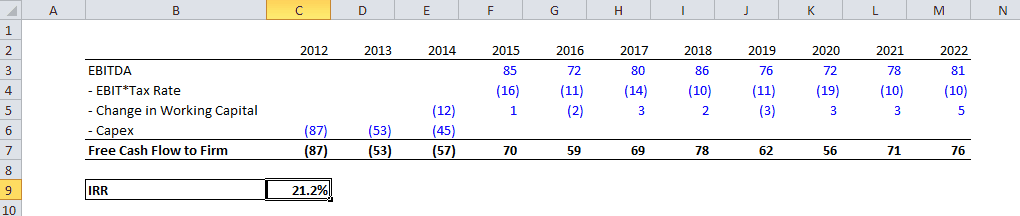top of page

## Finance Basics

Net Present Value

The net present value is a measure of the value creation of a project or an asset, this is achieved through the Discounted Cash Flow (DCF) methodology which consists in discounting future cash flows to calculate their present value. You can find here the methodology to discount cash flows.

To calculate the value or the value creation of an asset, you need to discount past, present and future Free Cash Flows to Firm (calculation here) using the same discount rate.Excel modeling

1. Calculate the FCFF, FCFE or Cash to Shareholders (details here)

2. Calculate the relevant discount rate

3. Calculate the discount factor for each year (details here)

4. Using the SUMPRODUCT function (see Excel functions here), multiply the Cash Flows with the discount factors

Never use the NPV function in Excel, it automatically assumes that the first value is one year from now and discounts it as such.The Internal Rate of Return (IRR)

The IRR is the discount rate that makes the NPV of a given series of cash flows equal to zero. If the discount rate is higher than the IRR, then the NPV will be negative, if it is lower than the IRR it will be positive.Excel modeling

1. Calculate the FCFF, FCFE or Cash to Shareholders (details here)

2. Use the IRR formula (or XIRR if your cash flows are not annual cash flows), select the range of the cash flows and indicate 0.1 in the guess field (this is the first value Excel will try, this should avoid any error)Pros and ConsNPV

• While only the NPV can estimate the value of an asset or the value created by a project, it is more fragile than the IRR as it relies on both the cash flow forecasts and on the discount rate applied to these cash flows

• The NPV also requires guidelines to determines as of what date is should be calculated

IRR

• The IRR is the most commonly used metric in the finance industry because it is easy to calculate and does not require any discount rate

• The IRR does not account for the duration of the project nor its size, for this reason it is not a suitable tool to discriminate projects or compare them

• There can be cases where there is no IRR or several IRRs

The example below shows why projects cannot be compared using the IRR.In the the example above, if the investment criteria is the IRR, project A will be selected despite being much small in size and shorter in duration.

bottom of page